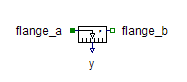Multiphase Voltage Sensor - MapleSim Help

Voltage Sensor

Sense multiphase voltage differencesDescription The Multiphase Voltage Sensor (or Voltage Sensor) component contains $m$ single-phase voltage sensor models, each connected between corresponding phases of ${\mathrm{plug}}_{p}$ and ${\mathrm{plug}}_{n}$, with the outputs connected to the corresponding signal of an output vector.Connections

 Name Description Modelica ID ${\mathrm{plug}}_{p}$ Positive $m$-phase plug plug_p ${\mathrm{plug}}_{n}$ Negative $m$-phase plug plug_n $v$ Real output $m$-vector; voltages in $V$ vParameters

 Name Default Units Description Modelica ID $m$ $3$ Number of phases mModelica Standard Library The component described in this topic is from the Modelica Standard Library. To view the original documentation, which includes author and copyright information, click here.